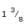# Races and Games - 2

## 10 Questions MCQ Test General Knowledge | Races and Games - 2

Description
Attempt Races and Games - 2 | 10 questions in 10 minutes | Mock test for Current Affairs preparation | Free important questions MCQ to study General Knowledge for Current Affairs Exam | Download free PDF with solutions
QUESTION: 1

Solution:
QUESTION: 2

Solution:
QUESTION: 3

### In a game of 100 points, A can give B 20 points and C 28 points. Then, B can give C:

Solution:
QUESTION: 4
In a 200 metres race A beats B by 35 m or 7 seconds. A's time over the course is:
Solution:
QUESTION: 5

A runstimes As fast as B. If A gives a start of 90m and they reach the goal at the same time.
The goal is at distance of:

Solution:
QUESTION: 6
In a 100 m race, A beats B by 10 m and C by 13 m. In a race of 180 m, B will beat C by:
Solution:
QUESTION: 7
In a game of 90 points A can give B 15 points and C 30 points. How many points can B give C in a game of 100 points?
Solution:
QUESTION: 8
A, B and C are the three contestants in one km race. If A can give B a start of 40 metres and A can give C a start of 64 metres. How many metres start can B give C?
Solution:
QUESTION: 9
P runs 1 km in 3 minutes and Q in 4 minutes 10 secs. How many metres start can P give Q in 1 kilometre race, so that the race may end in a dead heat?
Solution:
QUESTION: 10
In a 100 metres race. A runs at a speed of 2 metres per seconds. If A gives B a start of 4 metres and still beats him by 10 seconds, find the speed of B.
Solution:Use Code STAYHOME200 and get INR 200 additional OFF Use Coupon Code